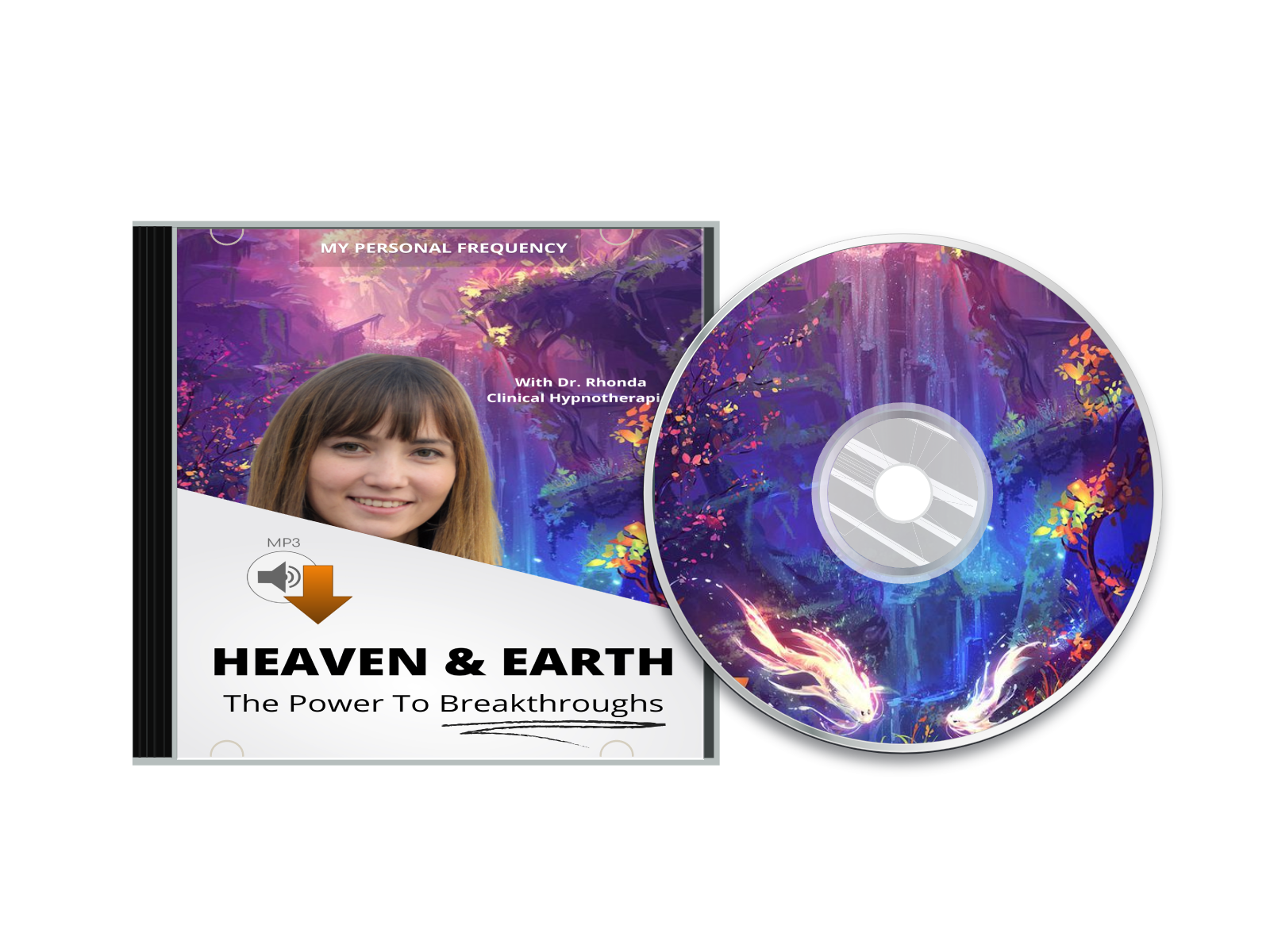"Heaven & Earth" contains powerful theta entrainment that sparkles light and positive vibes from above and ease your nervous system into a state of full relaxation.``` // Set the date we're counting down to var hihihi = new Date().getTime() + 5 * 60000; //var countDownDate = new Date("Jan 5, 2022 15:37:25").getTime(); var countDownDate = hihihi; // Update the count down every 1 second var x = setInterval(function() { // Get today's date and time var now = new Date().getTime(); // Find the distance between now and the count down date var distance = countDownDate - now; // Time calculations for days, hours, minutes and seconds var days = Math.floor(distance / (1000 * 60 * 60 * 24)); var hours = Math.floor((distance % (1000 * 60 * 60 * 24)) / (1000 * 60 * 60)); var minutes = Math.floor((distance % (1000 * 60 * 60)) / (1000 * 60)); var seconds = Math.floor((distance % (1000 * 60)) / 1000); // Output the result in an element with id="demo" //document.getElementById("demo").innerHTML = days + "d " + hours + "h " //+ minutes + "m " + seconds + "s "; document.getElementById("demo").innerHTML = minutes + "m " + seconds + "s "; // If the count down is over, write some text if (distance < 0) { clearInterval(x); document.getElementById("demo").innerHTML = "0m 00s"; } }, 1000); ```

Join Dr. Rhonda in this journey to help: